# Gage R&R (Repeatability & Reproducibility) - A Guide

### 01. What is a Gage R&R study?

In a perfect world, we would make every feature of every part at exactly the Nominal or Target design value. Unfortunately, in the real world, parts vary in size from the nominal or target value, and we expect to see differences in measured values from part-to-part.

But when me measure a set of parts, we do not know whether the gage is actually detecting differences in part sizes or whether the differences in measured value are caused by an unreliable gage or an untrained operator.

A Gage R&R study helps us answer the question: where does the variation come from?

• What fraction of this variation is truly coming from differences in part sizes (Part Variation)
• And what fraction of this variation is coming from the gage and operator?

For a measurement system to be considered reliable, most of the variation should come from differences in part sizes, with only a small fraction of variation coming from the gage and the operator. (Note: For the purpose of a Gage R&R study, we describe the measurement system as a combination of the gage, the operator, and the process by which the measurement is made.)

If a high fraction of variation is from the gage or the operator, i.e. the gage has poor R&R, parts that are close to the USL and LSL i.e. parts that fall in the yellow zone are at risk of being misclassified as Pass or Fail.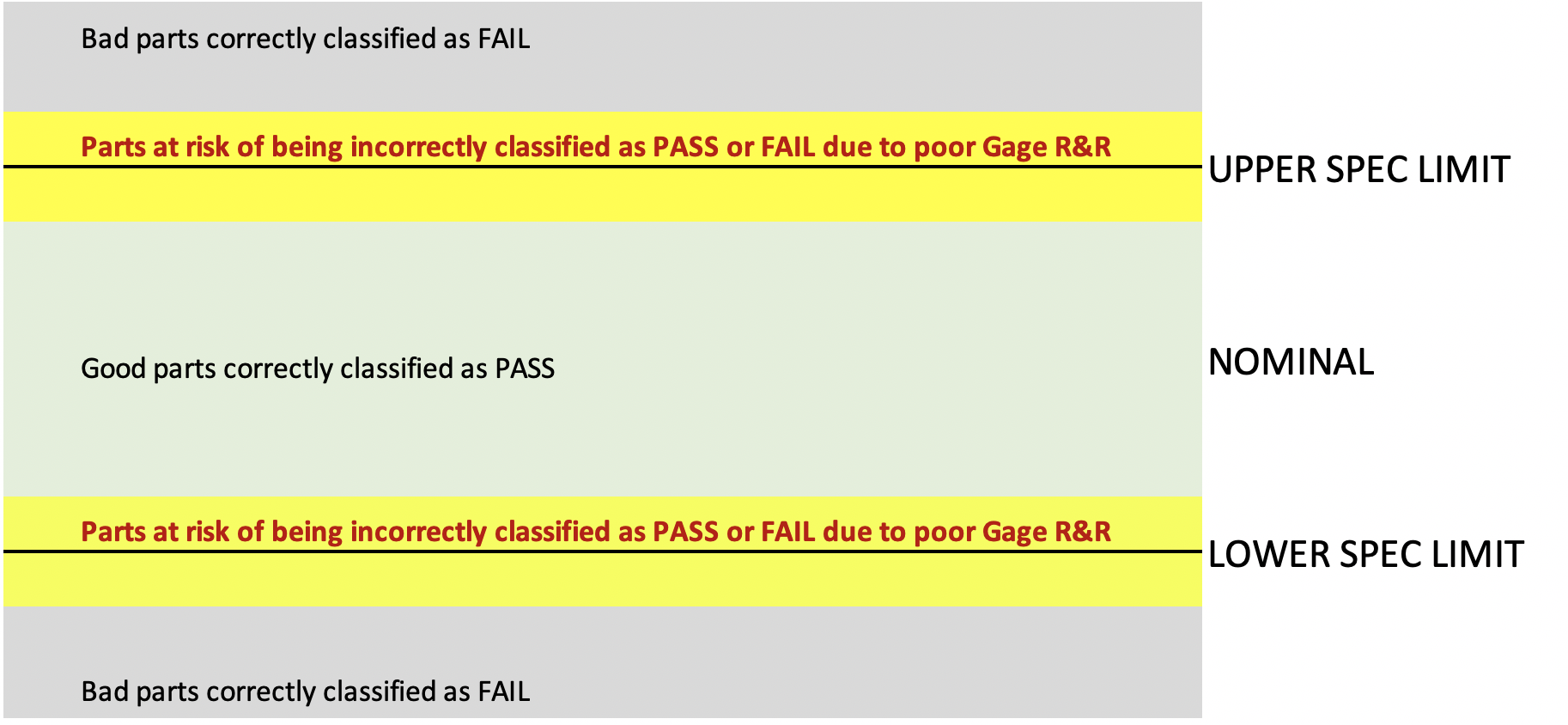### 02. An Analogy

As an example, you may choose to use an ear thermometer (the gage) to measure your child's body temperature (the parameter being measured). The measurement method involves inserting the tip of the ear thermometer into the ear canal, and then pressing a button to record the temperature.

When you use the thermometer, you assume that:

1. This method will detect any significant increase in the child's body temperature.
2. The measurements will be nearly identical if you repeat the measurement (within a short interval of time), i.e. measurements are Repeatable across multiple trials.
3. The results will be nearly identical if you and your spouse were to independently make this measurement, i.e. measurements are Reproducible across operators.

In the ideal case, if you and your spouse were to make measurements a few hours apart, the only reason for a change in reading should be an actual change in body temperature. How you position the thermometer or any inherent variability in the thermometer should have little to no impact on the measurement. And this is why we run a Gage R&R study - to determine whether the difference in readings comes from the thermometer itself, the operator (person making the measurement), or from the patient's temperature (ideally all the variation comes from the patient's actual temperature).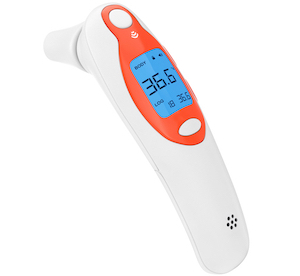### 03. Prerequisites

Before we dive into the mechanics of a Gage R&R study, let's define some important terms.

1. Bias: As a gage wears, we begin to see a consistent shift from the true value. The measured value will always be higher or lower than the actual value.
2. Linearity: Sometimes, the difference between measured value and the true value increases (or decreases) over the range of measurements. For example, when we measure a 1.000" block, we get an error of .0001" but when we measure a 2.000" block, we get an error of .00045". In this case the error increases as the size of the feature being measured increases.
3. Stability: Stability is the behavior of a measurement system over time. To ensure stability, we monitor environmental parameters such as Temperature and Humidity, and calibrate a gage periodically.
4. Calibration: To calibrate a gage, we measure a known value (e.g. a 1.000" block traceable to NIST) with the gage, and ensure that the reported value is within the acceptable tolerance range of the gage.

Before a Gage R&R study, we must ensure that the gage is unbiased, linear, stable, and calibrated.

### 04. Types of Gage R&R Studies

Gage R& R studies are broadly divided into two types depending on the type of measurement being made:

1. Attribute Gage R&R: Go/No-Go, Thread Gages, Visual Appraisal to a Standard etc.
2. Variable Gage R&R: Micrometers, Calipers, CMMs etc

Variable Gage R&R studies are further sub-classified into:

1. Crossed Gage R&R: In the most commonly used method, the study is crossed i.e., each operator measures each part or sample multiple times.
2. Nested Gage R&R: In some cases, it is not possible for each operator to measure each part or sample multiple times (e.g. destructive tests), requiring a nested study in which each operator measures a different set of parts (i.e. the parts are "nested" under the operator).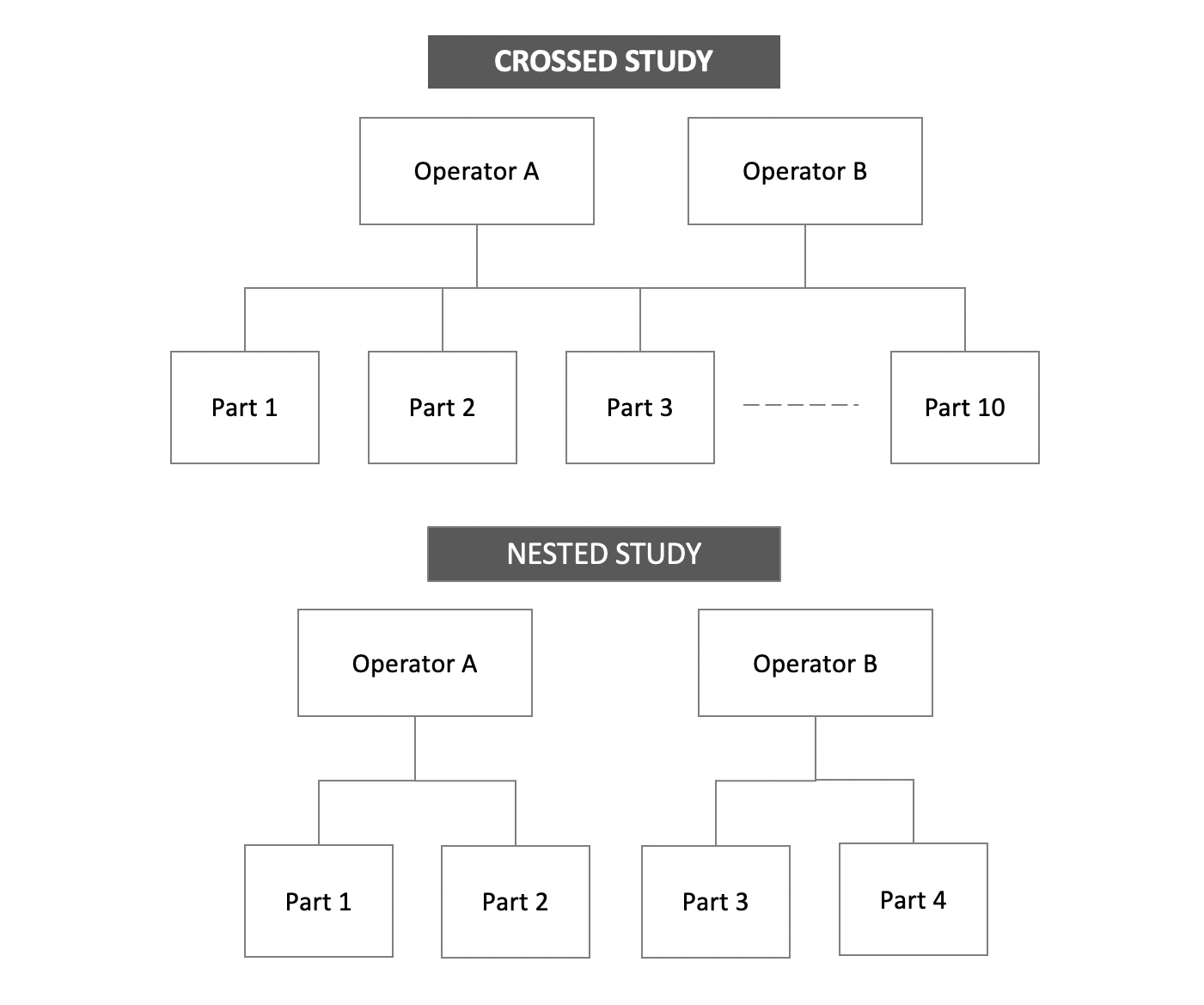### 05. Attribute Gage R&R Overview

A typical Attribute gage R&R study involves multiple operators, parts, and repeated measurements of the same part by each operator (we call these repeated measurements "trials"). It's common to have 3 Operators, 30 Parts, and 3 Trials on each part. Operators record a simple Pass/Fail (i.e. Attribute) measurement. (In more advanced cases, operators may need to classify parts into categories e.g. Class A, Class B, and Class C)

IMPORTANT: In an Attribute Gage R&R study, disagreement within each Operator's trials and disagreements across Operators will be apparent only in parts that are close to the Upper Spec Limit (USL) and Lower Spec Limit (LSL).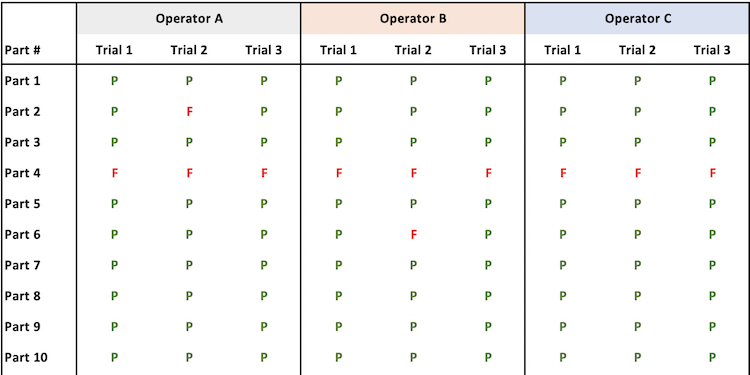### 06. Attribute Gage R&R Calculations

Each operator's appraisal (Pass/Fail) is compared to the appraisal (Pass/Fail) of the other two operators, and against a reference value. The reference value itself must be obtained from a reliable variable measurement system (e.g. a CMM, a color measurement device etc.) or from a master/expert appraiser.

Repeatability: For each operator, we measure how many times they arrive at the same assessment within each trial (i.e. are each operator's measurements for the same part consistent?). We then divide this number by the total number of parts to arrive at the percentage repeatability. If the operator's measurements on the same part are consistent less than 90% of the time, the operator needs re-training.

Reproducibility: Next we measure how many times the operators agree with each other's individual measurements (i.e. are measurements of the same part consistent across Operators?). We then divide this number by the total number of parts to arrive at percentage reproducibility.

### 07. Variable Gage R&R Overview

A typical Variable (crossed) Gage R&R involves multiple operators, parts, and repeated measurements of the same part by each operator (we call these repeated measurements "trials").

It's common to have 3 Operators (k), 10 Parts (n), and 3 Trials (r) on each part. Each operator measures each part three times, records the numeric (i.e. variable) measurement value, and we obtain a table like the one shown here.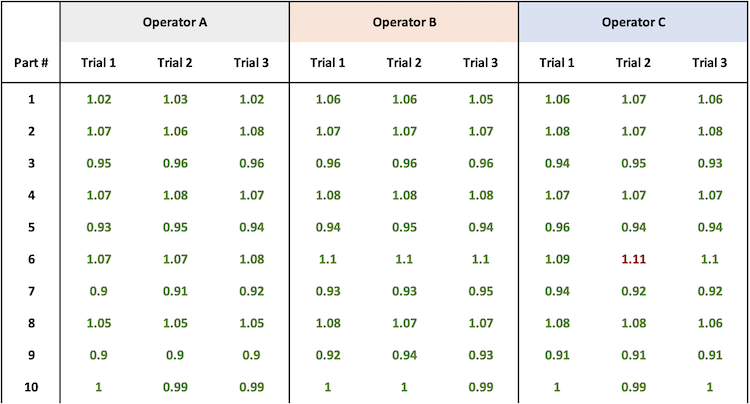### 08. ANOVA (Analysis of Variance)

The first step is to calculate the total variation. But once we have the total variation, how do we know what fraction of the variation comes from the parts themselves, and what fraction comes from the operator or the gage?

This is where the ANOVA (Analysis of Variance) technique comes handy. It allows us to partition the variation into factors.

Variability-Total = Variability-from-Parts + Variability-from-Operators + Variability-from-Equipment + Variability-from-Interaction-Between-Operator-And-Gage

SSTotal = SSParts + SSOperator + SSEquipment +SSInteraction

### 09. Total Variation

To calculate the total variation, we calculate the sum of the square of the difference between each measured value and the overall average. We call this the Total Sum of Squares (SS-Total).

For example:

• The Square Error for "Operator A - Part 1 - Trial 1" is (1.02 - 1.008)2 = (0.012)2 = .000144
• The Square Error for "Operator B - Part 8 - Trial 2" is (1.07 - 1.008)2 = (0.062)2= .003844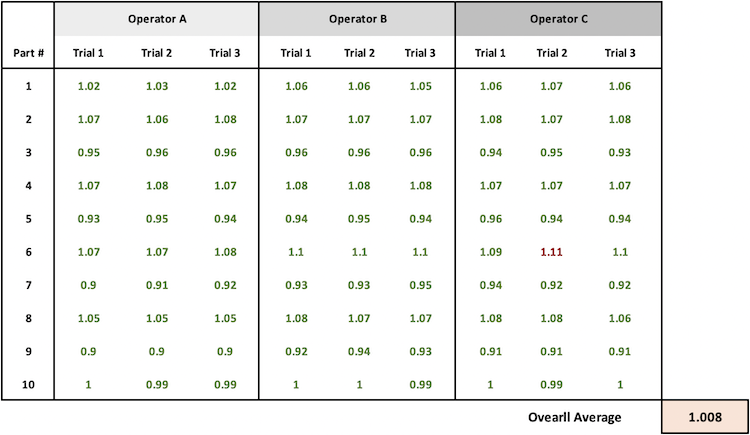### 10. ANOVA and Components of Variation

ANOVA uses a very simple trick to calculate the fraction of variability from Parts, Operators, and Equipment. We group the data set in different ways to examine whether the measurement differences are between parts (desired), or between operators (a reproducibility problem), or between trials (a repeatability problem).

There are four groupings of interest:

1. Grouping by Parts
2. Grouping by Operators (Reproducibility)
3. Grouping by Operator and Part i.e. by Equipment (Repeatability)

### 11. Grouping by Parts

Ideally, all variation comes from the parts themselves. So we expect to see a high amount of variation between parts. To determine how much variation comes from the parts, we group the data by part, and calculate the average for each Part. Then we compare each of these Part average to the overall average.

This is the sum of the square of the difference between each part average and the overall average. There are 10 parts, and we calculate 10 Square-Error terms that we sum to arrive at SS-Parts.

For example:

• The Square Error for Part 1 is (1.048 - 1.008)2 = (0.04)2 = .0016
• The Square Error for Part 7 is (.924 - 1.008)2 = (-0.084)2= .0070

Note the negative sign in the error calculation for Part 7. We square the error values to eliminate the negative sign. Then we add all the squared values.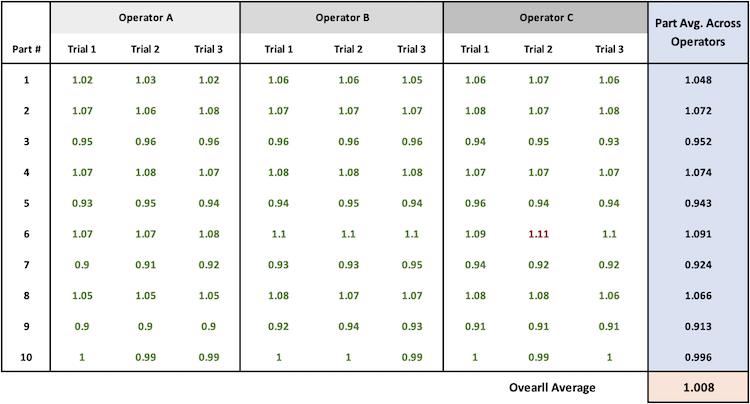### 12. Grouping by Operator (Reproducibility)

To determine how much variation comes from the operators, we group the data by Operator and calculate the mean for each Operator. Then we compare each of these Operator averages to the overall average.

This is the sum of the square of the difference between each operator average and the overall average. There are 3 operators, and we calculate 3 Square-Error terms that we sum to arrive at SS-Operators

• The Square Error for Operator A is (0.999 - 1.008)2 = (-0.009)2 = .000081
• The Square Error for Operator B is (1.015 - 1.008)2 = (.007)2= .000049
• The Square Error for Operator C is (1.010 - 1.008)2 = (.002)2= .000004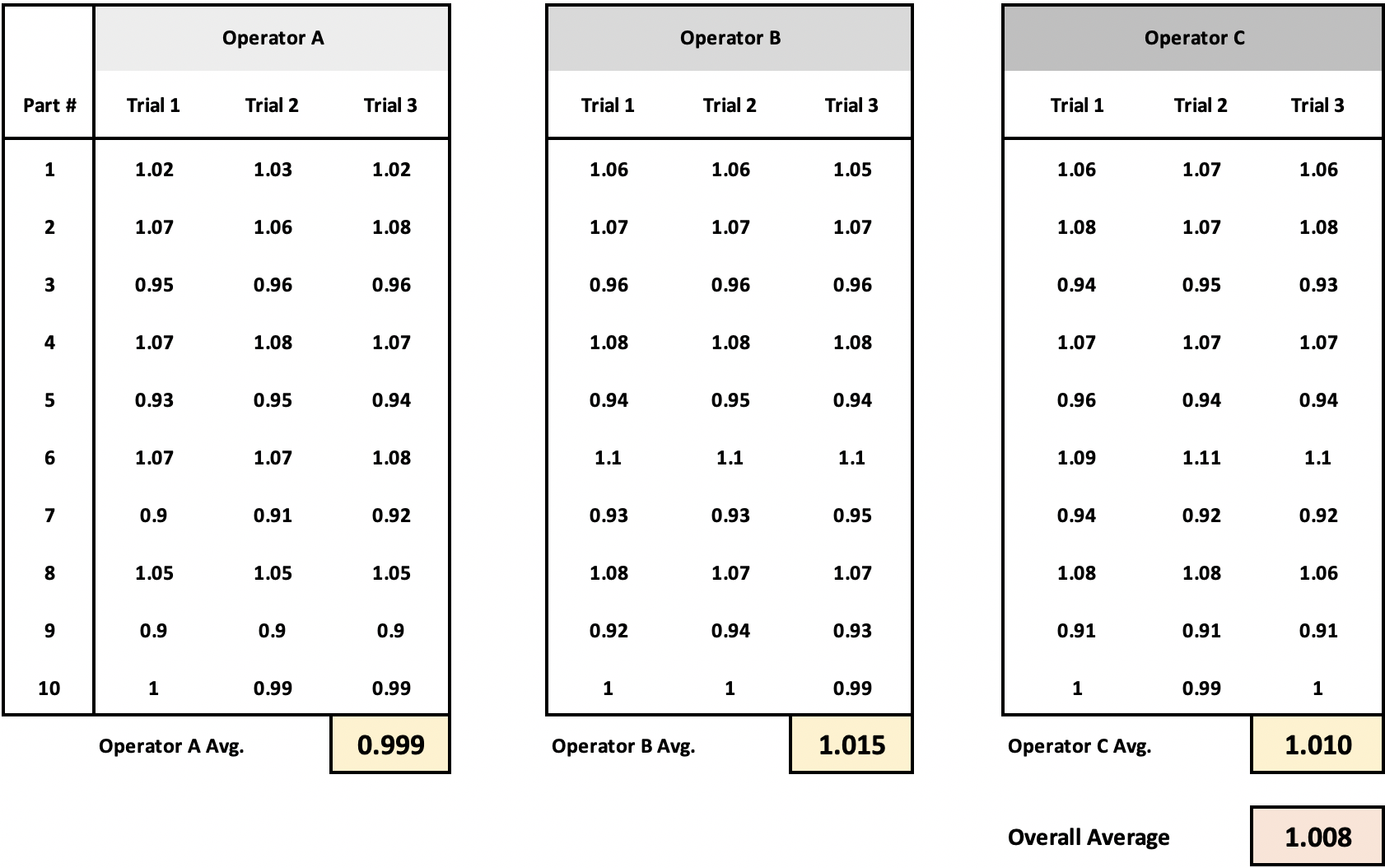### 13. Grouping by Operator and Part i.e. Equipment (Repeatability)

Quantifying the equipment measurement requires a different comparison. This time, to determine how much variation comes from the equipment, we group data by Operator and Part. This grouping gives us just the equipment variation because it is the same operator measuring the same part three times.

This is the sum of the square of the difference between each operator-part measurement and the corresponding operator-part average. There are 30 operator-part combinations, and we calculate 30 Square-Error terms that we sum to arrive at SS-Equipment

For example:

• The Square Error for "Operator A - Part 1 - Trial 1" is (1.02 - 1.023)2 = (-0.003)2= .000009
• The Square Error for "Operator A - Part 1 - Trial 2" is (1.03 - 1.023)2 = (-0.007)2= .000049
• The Square Error for "Operator A - Part 1 - Trial 3" is (1.02 - 1.023)2 = (-0.003)2= .000009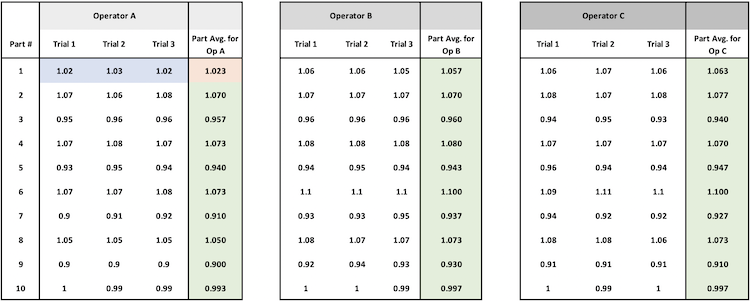### 14. Interaction between Operator and Gage i.e. Error Term

The interaction sum of squares is calculated by subtracting out the SSParts, SSOperator, and the SSEquipment from the SSTotal

SSInteraction = SSTotal - SSParts - SSOperator - SSEquipment